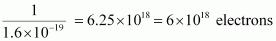Question 3

# Calculate the number of electrons constituting one coulomb of charge.

Charge is flow of number of electrons.

Q=ne

Q is charge, n = number, e = electron

Whereas, One electron possesses a charge of 1.6 × 10−19 C,

i.e., 1.6 × 10−19 C of charge is contained in 1 electron.

∴ 1 C of charge is contained inHence, 6x1018 electrons constitute one coulomb of charge.